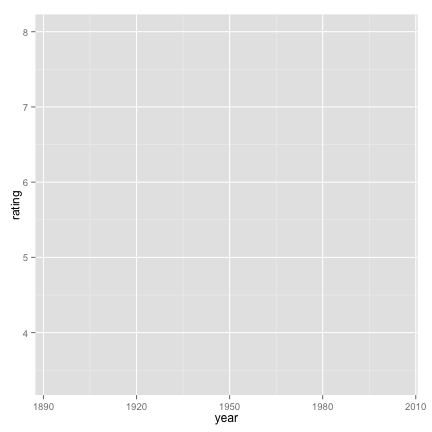# ggplot2: stat_summary()

``````## knitr configuration: http://yihui.name/knitr/options#chunk_options
opts_chunk\$set(comment = "", error= TRUE, warning = FALSE, message = FALSE,
tidy = FALSE, cache = F, echo = T,
fig.width = 6, fig.height = 6)

## R configuration
options(width = 116, scipen = 5)
``````

## References

``````library(ggplot2)
``````

## Summary

Summarizing the y values for each unique x value can be useful.

Currently, there is a bug (version 0.9.3) that prevents summary stat from working with line geom.

http://stackoverflow.com/questions/13775150/unusual-behavior-of-ggplot2-0-9-3

Even the ggplot2 web site has gone wrong.

http://docs.ggplot2.org/current/stat_summary.html

Summary function(s) should be given via these options

``````fun.data: Complete summary function. Should take data frame as input
and return data frame as output

fun.ymin: ymin summary function (should take numeric vector and return
single number)

fun.y: y summary function (should take numeric vector and return
single number)

fun.ymax: ymax summary function (should take numeric vector and return
single number)
``````

Functions to get multiple summary values

``````Wrap up a selection of summary functions from Hmisc to make it easy to use with 'stat_summary'.

Description:
See the Hmisc documentation for details of their options.

Usage:
mean_cl_boot(x, ...)
mean_cl_normal(x, ...)
mean_sdl(x, ...)
median_hilow(x, ...)
``````

Continuous X values

``````p.movies2 <- ggplot(data = movies,
mapping = aes(x = year, y = rating))

## median and line geom
p.movies2 + layer(geom = "line",
stat = "summary",
fun.y = median)
``````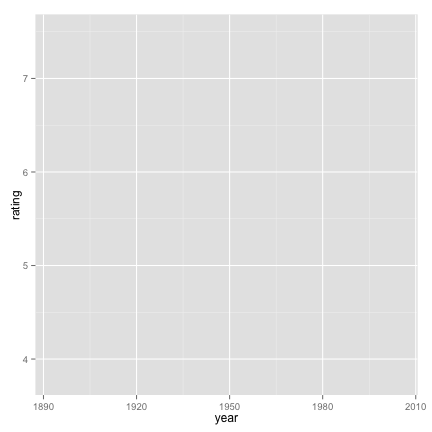``````## median_hilow and smooth geom
p.movies2 + layer(geom = "smooth",
stat = "summary",
fun.data = median_hilow)
``````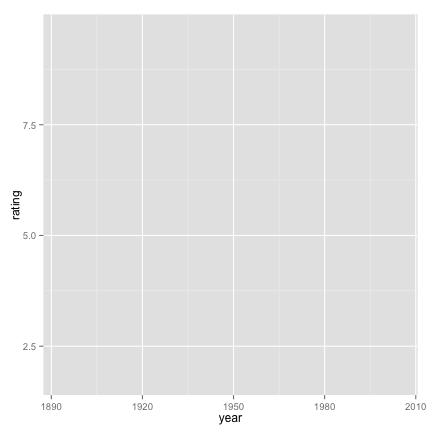``````## mean and line geom
p.movies2 + layer(geom = "line",
stat = "summary",
fun.y = mean)
``````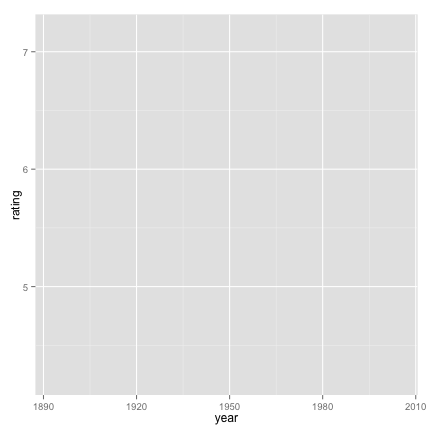``````## mean_cl_boot and smooth geom
p.movies2 + layer(geom = "smooth",
stat = "summary",
fun.data = mean_cl_boot)
``````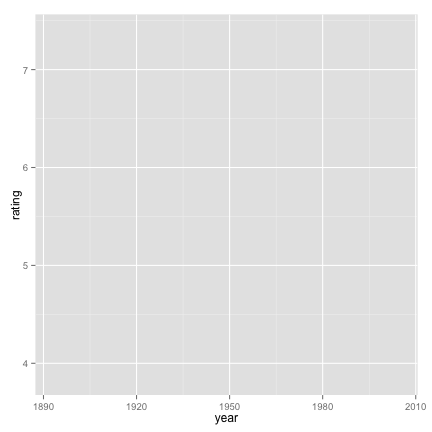Discrete X values

``````p.movies3 <- ggplot(data = movies,
mapping = aes(x = round(rating), y = log10(votes)))

## mean and point geom
p.movies3 + layer(geom = "point",
stat = "summary",
fun.y = mean)
``````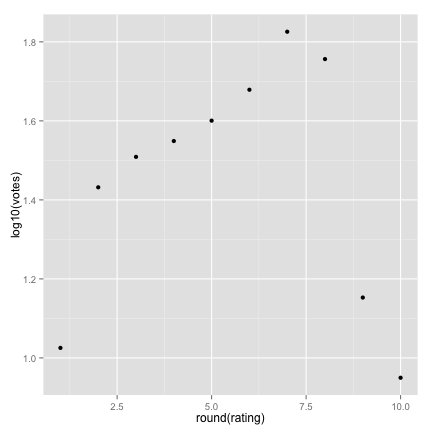``````## mean_cl_normal and errorbar geom
p.movies3 + layer(geom = "errorbar",
stat = "summary",
fun.data = mean_cl_normal)
``````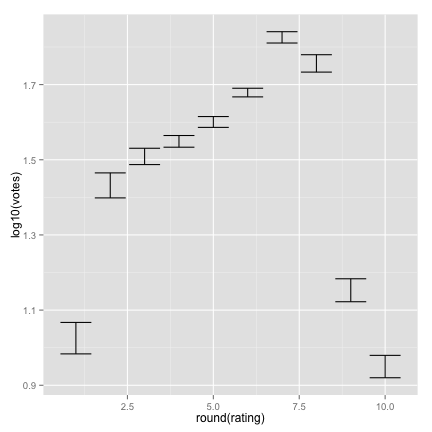``````## median_hilow and pointrange geom
p.movies3 + layer(geom = "pointrange",
stat = "summary",
fun.data = median_hilow)
``````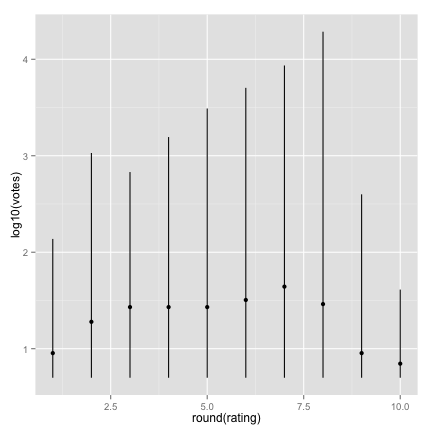``````## median_hilow and crossbar geom
p.movies3 + layer(geom = "crossbar",
stat = "summary",
fun.data = median_hilow)
``````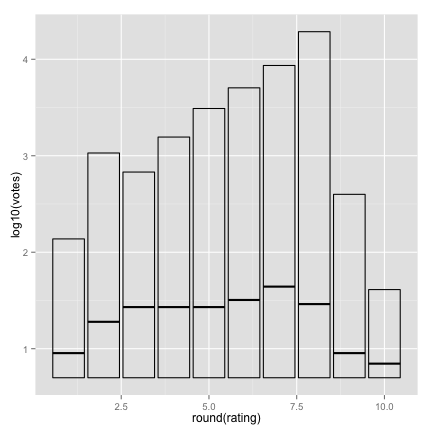``````## min/mean/max and crossbar geom
p.movies3 + layer(geom = "crossbar",
stat = "summary",
fun.y = mean, fun.ymax = max, fun.ymin = min)
``````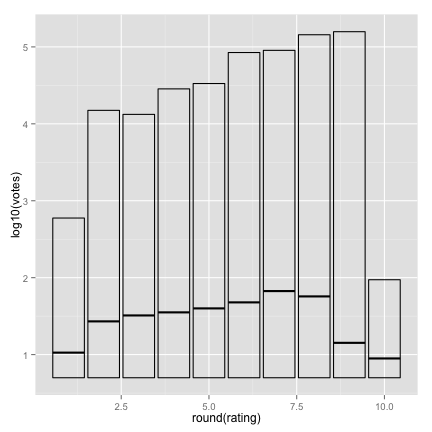Individual summary functions

``````midm <- function(x) mean(x, trim = 0.5)

p.movies3 +
layer(stat = "summary",
geom = "point",
mapping = aes(color = "trimmed"),
fun.y = midm
) +
layer(stat = "summary",
geom = "point",
mapping = aes(color = "raw"),
fun.y = mean
) +
scale_color_discrete(name = "Mean")
``````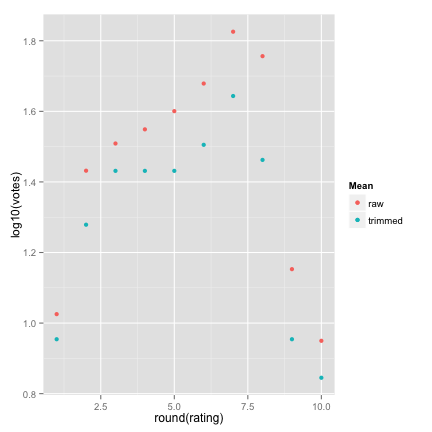Single summary function with multiple return values

This is not working either. stat_summary currently does not work with continuous X values.

``````iqr <- function(x, ...) {
qs <- quantile(as.numeric(x), probs = c(0.25, 0.75), na.rm = TRUE)
names(qs) <- c("ymin","ymax")
qs
}

p.movies2 + layer(stat = "summary",
geom = "ribbon",
fun.data = iqr)
``````